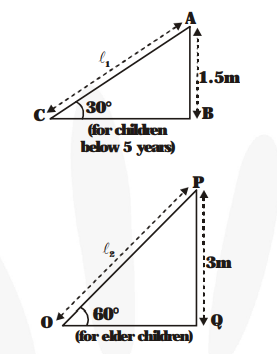# A contractor plans to install two slides for the children to play in a park.Question:

A contractor plans to install two slides for the children to play in a park. For the children below the age of 5 years, she perfers to have a slide whose top is at a height of 1.5 m and is inclinded at an angle of 30° to the ground, whereas for elder children, she wants to have a steep slide at a height of 3 m, and inclined at an angle of 60° to the ground. What should be the length of the slide in each case?

Solution:

In figure, $\ell_{1}$ is the length of the slide made for children below the age of 5 years and $\ell_{2}$ is the length of the slide made for elder children.In figure, $\mathrm{AB}=1.5 \mathrm{~m}, \mathrm{AC}=\ell_{1} \mathrm{~m}$ and $\angle \mathrm{ACB}=30^{\circ} ; \mathrm{PQ}=3 \mathrm{~m}, \mathrm{OP}=\ell_{2} \mathrm{~m}$ and $\angle \mathrm{POQ}=$ $60^{\circ}$

$\frac{A B}{A C}=\sin 30^{\circ} \quad$ and $\quad \frac{P Q}{O P}=\sin C 0^{\circ}$

$\Rightarrow \frac{\mathbf{1 . 5}}{\ell_{1}}=\frac{1}{\mathbf{2}} \quad$ and $\quad \frac{\mathbf{3}}{\ell_{2}}=\frac{\sqrt{\mathbf{3}}}{\mathbf{2}}$

$\Rightarrow \ell_{1}=2 \times 1.5 \mathrm{~m}$ and $\ell_{2}=\frac{\mathbf{3} \times \mathbf{2}}{\sqrt{\mathbf{3}}} \mathrm{m}$

$\omega=$ Final angular velocity